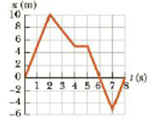Chapter 2, Problem 6P

Chapter
Section
Textbook Problem

A graph of position versus time for a certain particle moving along the x-axis is shown in Figure P2.6. Find the average velocity in the time intervals from (a) 0 to 2.00 s, (b) 0 to 4.00 s, (c) 2.00 s to 4.00 s, (d) 4.00 s to 7.00 s, and (e) 0 to 8.00 s.Figure P2.6 (Problems 6 and 17)

(a)

To determine
The average velocity of the particle in 0 to 2.00s .

Explanation

Given info: The initial position of the particle in the interval is 0 , the final position of the particle in the interval is 10.0m , the initial time of the interval is 0 , and the final time of the interval is 2.00s .

Explanation:

The formula used to calculate the average velocity is,

v¯=xfxitfti

Here,

v¯ is the average velocity.

xf is the final position.

xi is the initial position.

tf is the initial time.

ti is the final time.

Substitute 10.0m for xf , 0 for xi , 2

(b)

To determine
The average velocity of the particle in 0 to 4.00s .

(c)

To determine
The average velocity of the particle in 2.00s to 4.00s .

(d)

To determine
The average velocity of the particle in 4.00s to 7.00s .

(e)

To determine
The average velocity of the particle in 0 to 8.00s .

Still sussing out bartleby?

Check out a sample textbook solution.

See a sample solution

The Solution to Your Study Problems

Bartleby provides explanations to thousands of textbook problems written by our experts, many with advanced degrees!

Get Started

Find more solutions based on key concepts